# NCERT Solutions - Continuity & Differentiability, Exercise 5.4 JEE Notes | EduRev

## JEE : NCERT Solutions - Continuity & Differentiability, Exercise 5.4 JEE Notes | EduRev

The document NCERT Solutions - Continuity & Differentiability, Exercise 5.4 JEE Notes | EduRev is a part of the JEE Course Mathematics (Maths) Class 12.
All you need of JEE at this link: JEE

Continuity & Differentiability

Question 1:
Differentiate the following w.r.t. x: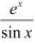Let y =By using the quotient rule, we obtain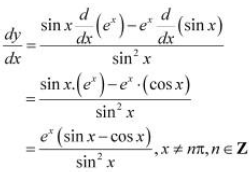Question 2: Differentiate the following w.r.t. x: esin-1x

Let y = esin-1x
By using the chain rule, we obtain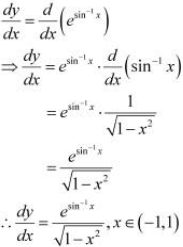Question 3: Differentiate the following w.r.t. x: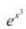Let y =By using the chain rule, we obtain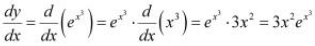Question 4: Differentiate the following w.r.t. x: sin(tan-1e-x)

Let y = sin(tan-1e-x)
By using the chain rule, we obtain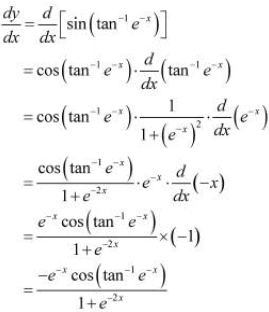Question 5: Differentiate the following w.r.t. x: log(cosex)

Let y = log(cosex)
By using the chain rule, we obtain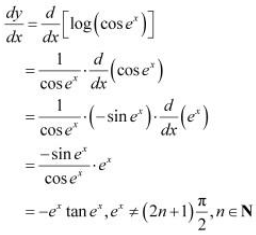Question 6: Differentiate the following w.r.t. x: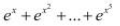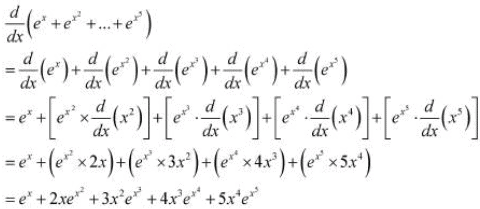Question 7: Differentiate the following w.r.t. x: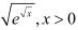Let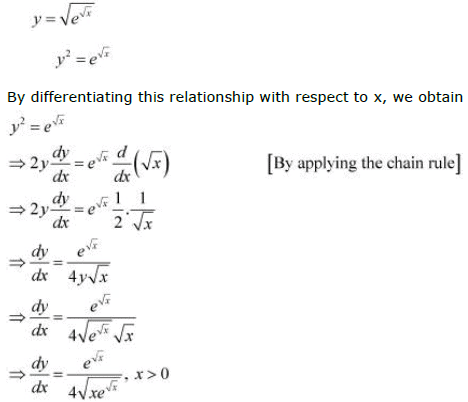Question 8: Differentiate the following w.r.t. x: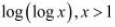Let y =By using the chain rule, we obtain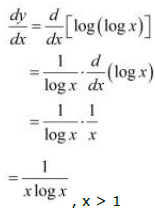Question 9: Differentiate the following w.r.t. x: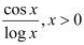Let y =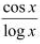By using the quotient rule, we obtain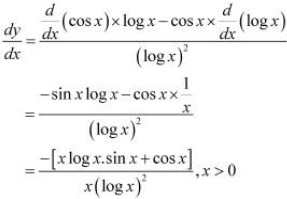Question 10: Differentiate the following w.r.t. x: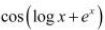, x>0

Let y =By using the chain rule, we obtain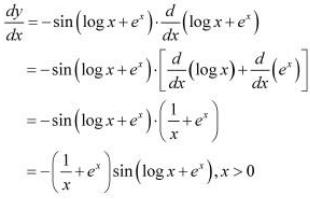Offer running on EduRev: Apply code STAYHOME200 to get INR 200 off on our premium plan EduRev Infinity!

## Mathematics (Maths) Class 12

209 videos|222 docs|124 tests

,

,

,

,

,

,

,

,

,

,

,

,

,

,

,

,

,

,

,

,

,

,

,

,

;